## Coming soon: Graphs, shape and divisionNext week I concentrate on resources for younger children.  Firstly, there is one of a superb set of year 2 maths worksheets which URBrainy.com have allowed me to publish. It is suitable for 6/7 year old children who are just beginning to understand and interpret block graphs and it looks at the favourite sandwich fillings of a class:- always a popular subject.

A pinboard is a great way to investigate shapes with young children. One can be made by nailing panel pins or small nails into a piece of plywood approximately 24cm × 24cm. I will be publishing a page which looks at how many different 3-sided shapes can be made with a pinboard. The results can be drawn onto the page of ‘pinboards’.

Year 3 children should be getting to grips with some of the easier ‘tables’. Once children have learnt the 2x, 4x, 5x and 10x tables they can put their knowledge to good use by working out the division questions on our division practice page.

## Year 5 Calculator game: Multiples of 9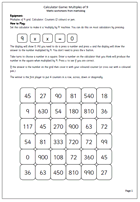The final game in our calculator series looking at tables and multiples. Once again the idea of the game is to get four counters in a row, this time on a multiples of 9 grid. Recognising multiples of 9 is relatively easy as the digits always add up to 9 or a multiple of 9. But knowing exactly which multiple is needed is much harder. Why not challenge your children?!!

Multiples of 9 calculator game

## Resource of the Week: Year 2 Investigation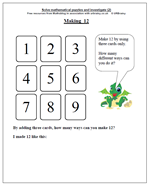Investigations are a great way to reinforce mathematical concepts and learn about patterns in maths. This maths worksheet is posted in our Year 2 section, under Using and Applying Maths and has proved to be one of our most used resources.

We have the digits from one to nine again, this time the task is to use three digits to make 12. This is much harder than just using two digits and it would be a good idea to have some cards printed out with the digits on to help with this. They are available in the reception maths worksheets section.

Again plenty of opportunity for talking about the numbers and what counts as a different way. Is 1 + 2 + 9 the same as 2 + 1 + 9 ?

Make 12

## Year 3 maths worksheet: Multiples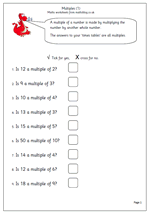It is in year 3 that the term ‘multiple’ is introduced. Whilst there are some very complex explanations of the term ‘multiple’ it is best thought of  as a number produced by multiplying a starter number by another whole number. The answers to ‘tables’ are all multiples.

These two Year 3 maths worksheets ask whether various numbers are multiples of others and it is interesting to see how children tackle these. With a good knowledge of tables they are quite easy. Without this knowledge of tables it can take an awfully long time. So, make sure those tables have been learned!

Multiples (1)

## Year 6 maths worksheet: Area of rectangles challenge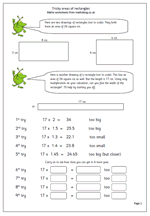This is a great worksheet for children to develop their ‘trial and improvement’ methods. The area of the rectangle is 24 sq cm. One side has a length of 17 cm. What must the other side measure? The catch is that only multiplication can be used to find the answer and a calculator is an absolute must!

The aim is to refine the answer closer and closer to the correct one, although this might never be reached! (No cheating by dividing 24 by 17, although this won’t give you a perfect answer!!) Some children become fascinated by this type of question and might need a calculator with a greater number of digits to work with.

Have a look at the page to get a more complete explanation and then see just how close you can get.

This can be found in our Year 6 measurement category.

Tricky rectangle areas

## Coming soon: area, multiples and multiplicationWe will be publishing some quite interesting worksheets next week. The first is a great worksheet for children to develop their ‘trial and improvement’ methods, suitable for year 6. The area of the rectangle is 24 sq cm. One side has a length of 17 cm. What must the other side measure? The catch is that only multiplication can be used to find the answer and a calculator is an absolute must! The aim is to refine the answer closer and closer to the correct one, although this might never be reached!

It is in year 3 that the term ‘multiple’ is introduced. I will be publishing two two Year 3 maths worksheets which ask whether various numbers are multiples of others and it is interesting to see how children tackle these.

The final game in our calculator series will look at tables and multiples. Once again the idea of the game is to get four counters in a row, this time on a multiples of 9 grid. Recognising multiples of 9 is relatively easy as the digits always add up to 9 or a multiple of 9. But knowing exactly which multiple is needed is much harder. Why not challenge your children?!!

## Year 3 maths worksheet: division and multiplication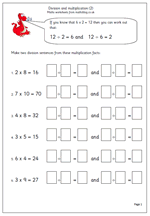This is the second page that looks at the relationship between division and multiplication and how knowledge of one fact can lead to knowing several other facts.

Some children fail to realise that division and multiplication are related, so when it comes to long division later, which involves mostly multiplication, they are lost.

This sheet shows clearly that if you know one multiplication fact then you can instantly work out two division facts. The only issue is that they use the numbers correctly ie if they know that 79 x 5 = 45 they alos know that 45 divided by 5 is 9 and that 45 divided by 9 is 5 (but it is obviously not true to say that 9 divided by 5 is 45!)

## Resource of the Week: Probability for Year 5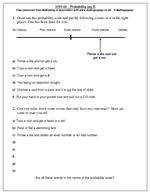Probably one of the least understood of maths concepts, especially with young children.

Children should be able to say whether events are impossible , unlikely , likely or certain.

They should also be able to say which events have an even chance of happening (such as tossing a coin and getting a head), but they should be careful not to say that if there are two possibilities, they are equally likely. For example, there are two possibilities – I might buy a new BMW today or I might not. Unfortunately, these two events are not equally likely. Another example of this is I choose a number between 1 and 5. Is the number I choose a prime number? As there are three prime numbers between 1 and 5 (2, 3 and 5) and two numbers that are not, there is not an even chance that I will choose a prime number.

Sometimes further investigations have to be carried out before a probability question can be answered. Take the possibility of a factor of 16 being even. The only way of doing this is to first find all the factors of 16, group them into even or odd and then work out the probability.

Free Y5 maths worksheet: Probability (pg 3)

## Year 4 maths worksheet: More dividing by 10 and 100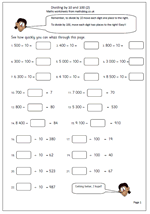This page looks for a rapid response from children to show that they clearly understand what is happening when numbers become 10 times or 100 times smaller.

The process is simple: to divide by 10 move each digit one place to the right. To divide by 100 move each digit 2 places to the right.

All the numbers are multiples of 10 so there is no need to get involved with decimal points, but some children might ask what happens to the zero. For example 500 divided by 10: move each digit one place to the right gives 50.0; this is correct but it can be written as 50.

Divide by 10 and 100 (2)

## Year 6 maths worksheet: Division with decimals (2)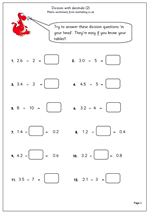This is the second of our pages on dividing decimals mentally. Concentrating on just one decimal place it is a good test of how well children know their tables and understand place value.

This is published in our Year 6 maths worksheets, under Knowing Number Facts. As well as these resources further number worksheets can also be found in or Four Rules section.

Divide decimals (2)